Fit Analyses

## Smoother Generalized Cross Validation

With the degrees of freedom of an estimate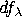,the mean squared error is given as
Cross-validation (CV) estimates the response at each xi from the smoother that uses only the remaining n-1 observations. The resulting cross validation mean squared error is
where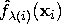is the fitted value at xi computed without the ith observation.

The cross validation mean squared error can also be written as
where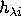is the ith diagonal element of the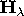matrix (Hastie and Tibshirani 1990).

Generalized cross validation replacesby its average value,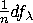.The generalized cross validation mean squared error is
Note The function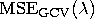may have multiple minima, so the value estimated by SAS/INSIGHT software may be only a local minimum, not the global minimum.